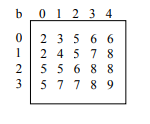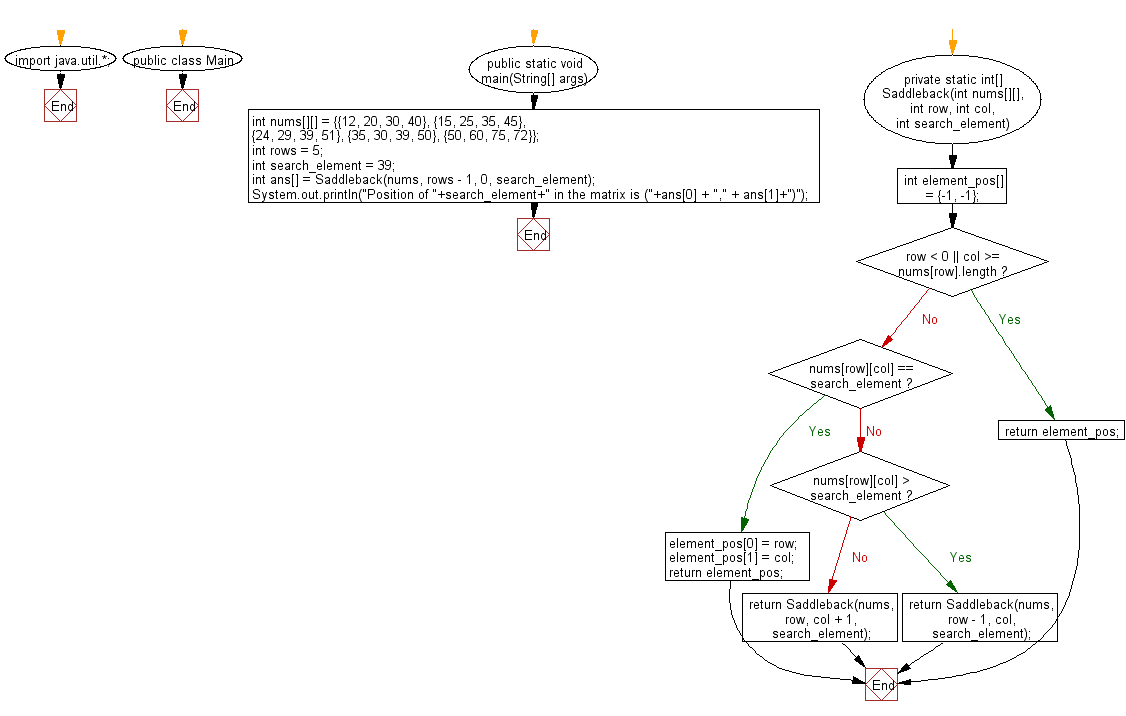﻿ Java exercises: Find the row, column position of a specified number in a given 2-dimensional array - w3resource# Java Exercises: Find the row, column position of a specified number in a given 2-dimensional array

## Java Search: Exercise-7 with Solution

Given is a 2-dimensional integer array [0..m-1, 0..n-1], each row and column of which is in ascending order (see example).

Example:Write a Java program to find the row, column position of a specified number (row, column position) in a given 2-dimensional array.

Sample Solution:

Java Code:

``````import java.util.*;
public class abc {

public static void main(String[] args) {
int nums[][] = {{12, 20, 30, 40},
{15, 25, 35, 45},
{24, 29, 39, 51},
{35, 30, 39, 50},
{50, 60, 75, 72}};

int rows = 5;
int search_element = 39;
int ans[] = Saddleback(nums, rows - 1, 0, search_element);
System.out.println("Position of "+search_element+" in the matrix is ("+ans + "," + ans+")");
}

/**
* @param nums, the matrix.
* @param row the current row.
* @param col the current column.
* @param search_element  the element that we want to search for.
* @return value: If found the index(row and column) of the element.
*  else return (-1 -1).
*/
private static int[] Saddleback(int nums[][], int row, int col, int search_element) {

//numsay to store the row and column of the searched element
int element_pos[] = {-1, -1};
if (row < 0 || col >= nums[row].length) {
return element_pos;
}
if (nums[row][col] == search_element) {
element_pos = row;
element_pos = col;
return element_pos;
}
//move up if the current element is greater than the given element
else if (nums[row][col] > search_element) {
return Saddleback(nums, row - 1, col, search_element);
}
//otherwise move right
return Saddleback(nums, row, col + 1, search_element);
}

/**
* Main method
*
* @param args Command line arguments
*/

}
```
```

Sample Output:

```Position of 39 in the matrix is (3,2)
```

Flowchart:Java Code Editor:

What is the difficulty level of this exercise?

﻿

## Java: Tips of the Day

Sound reproduction:

Sound is an important component of many applications, such as games. The Java programming language provides the means to work with it.

```import java.io.*;
import java.net.URL;
import javax.sound.sampled.*;
import javax.swing.*;

public class playSoundDemo extends JFrame {

// constructor
public playSoundDemo() {
this.setDefaultCloseOperation(JFrame.EXIT_ON_CLOSE);
this.setTitle("Play Sound Demo");
this.setSize(300, 200);
this.setVisible(true);

try {
URL url = this.getClass().getResource("MyAudio.wav");
AudioInputStream audioIn = AudioSystem.getAudioInputStream(url);
Clip clip = AudioSystem.getClip();
clip.open(audioIn);
clip.start();
} catch (UnsupportedAudioFileException e) {
e.printStackTrace();
} catch (IOException e) {
e.printStackTrace();
} catch (LineUnavailableException e) {
e.printStackTrace();
}
}

public static void main(String[] args) {
new playSoundDemo();
}
}

```